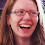## Tuesday, November 5, 2013

### Exploring Sine and Cosine Derivatives

My onwards saga with differential calculus presented via explorations! (See previous post here.)

My kids recently did an exploration of sine and cosine derivatives. It was a little long, but I think well worth it. The idea is this:

* First, we spent a class period reviewing how to find a sine or cosine equation given a graph. This way I made sure that they'd have the pre-requisite exploration algebra skills.

* On the worksheet, they were given f(x) = sin(x) and asked to sketch its derivative using their knowledge of how to sketch derivative graphs. They're quite confident with this sketch, and then they decided that f'(x) = cos(x) by looking at its shape. I circulated and made sure that each kid used the dy/dx feature of their calculator to verify that the amplitude of f' is still 1, by making sure that the steepest part of f has a derivative value of 1.

* They then are given g(x) = cos(x), and again they started by sketching its derivative graph in order to determine that g'(x) = -sin(x) by inspection.

* They then made predictions for when the amplitude of the original function is not 1. In each case, they sketched the curve and then verified the max derivative value, in order to verify the amplitude of their resulting derivative graph.

*  They repeated this for simple cases when the period changes. Based on their sketch of the derivative graph, they determined that the resulting derivative function would share the same period as the original functions, so they think k(x) = sin(2x) would have k'(x) = cos(2x). But, when they then checked the max derivative value along k(x), they realized that they were not correct. --WHY?? One of the kids immediately figured it out. I overheard him saying that because "sin(2x) is squished horizontally, that middle part of maximum steepness now becomes twice as steep as before, so obviously the amplitude will change." They checked this again using L(x) = cos(2x), whose max derivative (or steepness) is again 2, not 1, due to the horizontal compression. Brilliant!!

* They then tested their hypothesis out with M(x) = 3sin(2x), whose max steepness is now even steeper than 2.

* As a class, when we came together to discuss these exploratory problems together, I pulled up GeoGebra to guide our discussion. I entered something like M(x) = 3sin(2x) and had kids hypothesize just how steep that graph gets at x=0, since not everyone had quite reached the same conclusions before. If they said that the max derivative is 1, I entered y = 1x to show them that's unfortunately less steep than our curve is at x = 0. If they said 2, I entered y = 2x to show it's still less steep than our curve. Someone then guessed that the slope of the tangent at that point is 6, and we graphed it to see that y = 6x is indeed as steep as the curve gets. We then typed in M'(x) into the GeoGebra input bar, which automatically generates the formula AND the graph for the derivative. We discussed why the amplitude changes to 6, but why the period stays the same as that of M(x).

BAM! My kids are awesome!! They're able to now differentiate basic wave equations without knowing the first thing about chain rule. (Not yet, that's next, after we reinforce some other parts of our abstract analysis.) And they can explain the outcome of these sine and cosine derivative graphs! Yeah!!

1.What a super-cool approach to this idea. I especially appreciate how you were able to check student ideas against the slopes of linear equations using GeoGebra. I always like giving students a tool to verify their results that isn't "ask the teacher."

I just did a self-checking activity with students in class today and several of them still asked me to verify their answers. Argh!

2.Thank you!!! I try to encourage kids to ask me, "How can I check this using the calculator?" instead of "Is this right?" Now that we're two months into the year, they're getting much better about it! Slowly but steadily.

3.Great idea! Do they use TI84s or Desmos, or something else?. Although I've taught calculus for four years, I've never really mastered what the calculator can do to help me.

4.They generally use their graphing calcs to self monitor derived results or to check eqn equivalence. I use Geogebra regularly as visual demo at board. They've only used it a couple of times so far, but will be using it more later for projects and such.

1.For example, if i give them two consecutive turning points on a wave, they would write the wave eqn, CHECK via Trace (x= feature) on the calc that it passes both turning points, before differentiating and graphing the derivative function on top of original function and visually or numerically double checking their corresponding values/shape.